## A certain semiconductor device requires a tunneling probability of T = 10-5 for an electron tunneling through a rectangular barrier with a b

Question

A certain semiconductor device requires a tunneling probability of T = 10-5 for an electron tunneling through a rectangular barrier with a barrier height of Vo = 0.4 eV, the electron energy is 0.04 eV Determine the maximum barrier width.

in progress 0
1 month 2021-07-31T19:38:29+00:00 1 Answers 2 views 0

Generally the barrier width is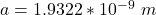Step-by-step explanation:

From the question we are told that

The tunneling probability required is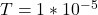The barrier height is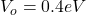The electron energy is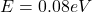Generally the wave number is mathematically represented as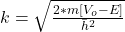Here m is the mass of the electron with the value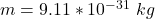h  is is know as h-bar and the value is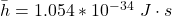So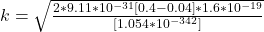=>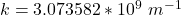Generally the tunneling probability is mathematically represented as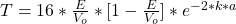So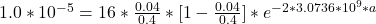=>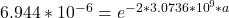Taking natural log of both sides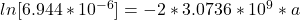=>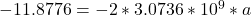=>